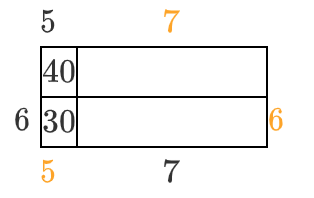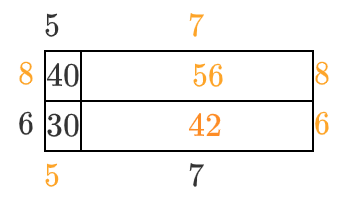Home > CC3MN > Chapter 2 > Lesson 2.1.4 > Problem2-45

2-45.

Find the perimeter of the entire rectangle shown at right (that is, the length of the outside boundary of the figure).  Notice that the areas of two of the parts have been labeled inside the rectangle.  Also find the total area.  Remember to show all work leading to your solution.

 Generic rectangle top edge left $5$ top edge right blank left edge top blank interior top left $40$ interior top right blank left edge bottom $6$ interior bottom left $30$ interior bottom right blank bottom left edge blank bottom right edge $7$

Remember that the numbers within the boxes are the products of each box's dimensions. Using that information, attempt to find the remaining dimensions of the rectangle.

Because the shape is a rectangle by definition, the opposite sides are equal in length.

Using the information given above, label the parts of the rectangle that can be found just by looking at the shape. Additions to the generic rectangle as follows: Top edge right is 7. Right edge bottom is 6. Bottom edge left is 5.

Now find the pieces not given.
$5\left( ? \right) = 40$
$6 \times 7 = 42$
$8 \times 7 = 56$

Additions to the generic rectangle as follows: Left edge top and right edge top, each is 8. Interior top right is 56. Interior bottom right is 42.Find the perimeter and area using the strategy shown in problem 2-8.

$\text{perimeter }= 52 \text{ units}$
$\text{area }= 168 \text{ units}^{2}$